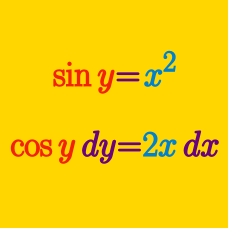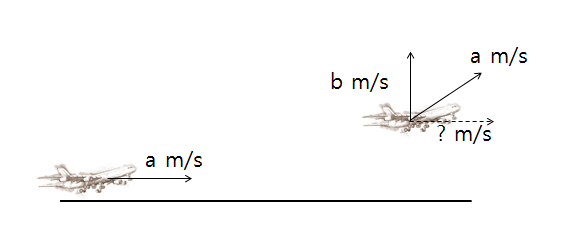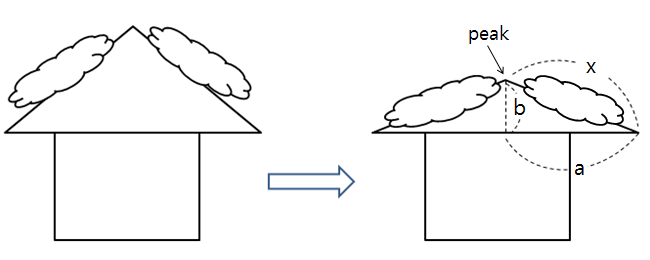Calculus

# Implicit Differentiation - Related Rates

In a fairy tale, a wizard rides a cloud which is moving to the right at a speed of $15\text{ m/s.}$ The wizard throws a ball vertically upward with a speed of $3\text{ m/s}$ relative to the cloud. When the cloud has moved to the right $30 \text{ m}$ following the throw, what is the velocity of the ball as seen by people on the ground?

Assume that air resistance is negligible and ignore the effect of gravity.As shown in the above diagram, a plane taxis off a runway at a speed of $a \text{ m/s} = 42 \text{ m/s}$ in order to take off. After taking off, the plane continues flying at $42 \text{ m/s}$, with vertical speed $b \text{ m/s} = 24 \text{ m/s}.$ What is the horizontal speed of the plane $5$ seconds after take-off?

Two people $A$ and $B$ start off at the point $(0,0)$ at the same time and walk east and north at $10\text{ m/min}$ and $6\text{ m/min},$ respectively. What is the rate at which their distance increases after $3\text{ min}?$As shown in the above digram, a thick layer of snow accumulates on a vertically symmetrical peaked roof, which causes the roof to slowly collapse downward under the weight of the snow. The length of the peaked roof is $x=28 \text{ m}$ on each side and the length of the base of the roof is $2a.$ If the collapse of the roof is such that $a$ increases at a rate of $5$ meters per hour, how fast is the height $b$ of the roof collapsing when $a=18?$

Note: The above diagram is not drawn to scale.

An aircraft is flying 8000 feet above the ground. It is moving due east at a velocity of 900 feet per second and is located due east of an observation tower. At a certain moment, the angle of elevation is $\frac{ \pi}{4}$. At that time, what is the rate of increase of the angle of elevation (in radians per second)?

×